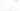## Logistic Regression: Cost Function

We cannot use the same cost function that we use for linear regression because the Logistic (Sigmoid) Function will cause the output to be wavy, causing many local optima. In other words, it will not be a convex function.Instead, our cost function for logistic regression looks like:

\begin{aligned} &J(\theta) = \dfrac{1}{m} \sum_{i=1}^m \mathrm{Cost}(h_\theta(x^{(i)}),y^{(i)}) \\ &\mathrm{where} \\ &\mathrm{Cost}(h_\theta(x),y) = \begin{cases} -\log(h_\theta(x)) & \text{if } y = 1 \\ -\log(1-h_\theta(x)) & \text{if } y = 0 \end{cases} \end{aligned}

Note that writing the cost function in this way guarantees that $J(\theta)$ is convex for logistic regression.

$\mathrm{Cost}(h_\theta(x),y)$ actually gives us the cost for a particular training example $(x,y)$ at a particular $\theta$. Plotting $\mathrm{Cost}(h_\theta(x),y)$ w.r.t. $h_\theta(x)$ gives:\begin{aligned} & \mathrm{Cost}(h_\theta(x),y) = 0 \space \space \space \text{ if } h_\theta(x) = y \\ & \mathrm{Cost}(h_\theta(x),y) \rightarrow \infty \begin{cases} \text{ if } y = 0 \; \mathrm{and} \; h_\theta(x) \rightarrow 1 \\ \text{ if } y = 1 \; \mathrm{and} \; h_\theta(x) \rightarrow 0 \end{cases} \end{aligned}